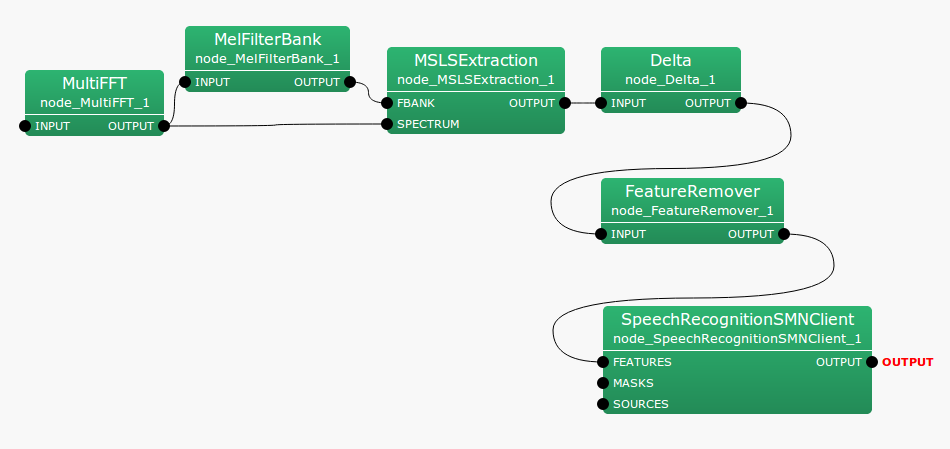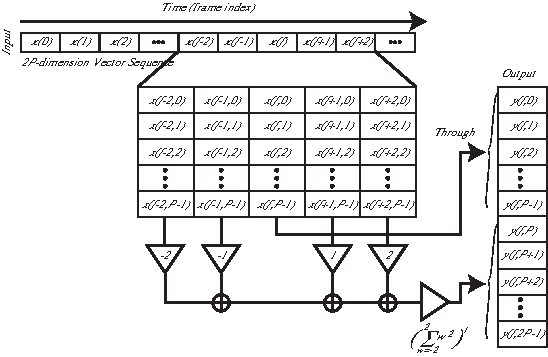## 6.4.1 Delta

### 6.4.1.1 Outline of the node

This node acquires dynamic feature vectors from static feature vectors. It is usually connected to the posterior half of MSLSExtraction  and MFCCExtraction , which are feature extraction nodes. These feature-extracting nodes acquire static feature vectors while reserving the regions where dynamic features are saved. The dynamical feature of this time is set to 0. The Delta  node calculates dynamic feature vector values with static feature vector values and set the values. Therefore, dimension numbers are same at the input and output.

### 6.4.1.2 Necessary file

No files are required.

### 6.4.1.3 Usage

When to use

This node is used for obtaining dynamic features from static features. It is usually used after MFCCExtraction  and MSLSExtraction .

Typical connectionFigure 6.82: Typical connection example for Delta

### 6.4.1.4 Input-output and property of the node

Table 6.76: Parameter list of Delta
 Parameter name Type Default value Unit Description FBANK_COUNT 13 Dimension number of static feature
INPUT

: Map<int, ObjectRef> type. A pair of the sound source ID and feature vector as Vector<float>  type data.

Output

OUTPUT

: Map<int, ObjectRef> type. A pair of the sound source ID and feature vector as Vector<float>  type data.

Parameter

FBANK_COUNT

: int  type. Dimension numbers of features to be processed. Its range is positive integers. When connecting it just after the feature extract node, the same FBANK_COUNT used in feature extraction. However, in the case that true is selected for the option used for the power term in feature extraction, set FBANK_COUNT +1.

### 6.4.1.5 Details of the node

This node obtains dynamic feature vectors from static feature vectors. The dimension number of inputs is the total dimension number of dynamic and static features. Dynamic features are calculated with an assumption that the dimension elements less than FBANK_COUNT are static features. Dynamic features are added to the dimension elements higher than FBANK_COUNT. The input feature vector at the frame time $f$ is expressed as follows.

 $\displaystyle \boldsymbol {x}(f)$ $\displaystyle =$ $\displaystyle [x(f,0),x(f,1),\dots ,x(f,P-1)]^{T}$ (137)

Here, $P$ is FBANK_COUNT.

 $\displaystyle \boldsymbol {y}(f)$ $\displaystyle =$ $\displaystyle [x(f,0),x(f,1),\dots ,x(f,2P-1)]^{T}$ (138)

Each output vector element is expressed as,

 $\displaystyle y(f,p)$ $\displaystyle =$ $\displaystyle \left\{ \begin{array}{ll} x(f,p), & {if~ ~ } p=0, \dots , P-1, \\ \displaystyle w \sum _{\tau =-2}^{2} \tau \cdot x(f+\tau ,p), & {if~ ~ } p=P, \dots , 2P-1, \end{array} \right.$ (139)

Here, $w = \frac{1}{\sum _{\tau =-2}^{\tau =2} \tau ^2}$. Figure 6.83 shows the input-output flow of Delta .Figure 6.83: Input-output flow of Delta .